# Implantable Sensor with Lamp (A3037)

© 2020 Kevan Hashemi, Open Source Instruments Inc.

## Contents

Description
Versions
Design
Set-Up
Battery Capacity
Optical Power
Stimulator Isolation
Modifications
Development

## Description

[10-FEB-20] The Implantable Sesor with Lamp (A3037) is a wireless electrical stimulator and biopotential sensor. It receives radio-frequency commands and transmits radio-frequency data messages. Its transmissions include biopotential samples, acknowledgements, and battery measurements. The displacement volume of the Mouse-Sized ISL (A3036A) will be no more than 1.3 ml. The electrical stimulus is delivered through two silicone-insulated helical wires terminated with miniature pins. A single radio-frequency command initiates an arbitrarily long stimulus. When combined with the A3036IL series of implantable lamps, the A3037 provides optogenetic stimulus. When combined with a bipolar depth electrode, the A3037 provides direct electrical stimulus.

The A3037 is powered by two separate batteries. One battery provides power to command reception, amplifiers, converters, and transmission circuits. The other provides the stimulus power. We call these the master and the stimulator circuits respectively. The master sends the stimulus control signal to the stimulator circuirt by means of an isolator. The isolator makes sure there is no electrical connection between the two circuits at frequencies lower than 1 kHz. This isolation stops current flowing from the stimulator into the sensor amplifier, helping to eliminate stimulation artifacts.

The master's battery is a manganese-lithium (MnLi) coin cell, while the switch's battery is a lithium-polymer (LiPo) pack. We can recharge both batteries through the A3037's sensor and stimulus leads with the help of two Battery Chargers (A3033).

Acknowledgement: The Mouse-Sized ISL development is funded by an SBIR grant from the NIH.

## Versions

The table below lists the existing versions of the Implantable Stimulator-Transponder (A3036).

Version Master Battery
Capacity
(mA-hr)
Stimulator Battery
Capacity
(mA-hr)
Volume
(ml)
A3037A 17 10 1.3 45 56
Table: Versions of the Implantable Sensor with Lamp (A3037).

The gold-plated pins on the end of the IST's lamp leads mate with a pair of sockets on the A3036IL implantable lamps.

## Design

The IST is managed by a field-programmable gate array (FPGA) in a 2.5-mm square package, the XO2-1200. This device provides both volatile and non-volatile memory as well as thousands of programmable logic gates. It is capable of implementing arbitrarily-complex stimuli in response to a single command. The A3037A uses the same firmware as the Implantable Sensor with Lamp (A3030E), in which a single stimulus consists of a set number of pulses, each of fixed length, generated at regular intervales, or at random intervals with a known average value.

## Set-Up

The figure below shows how the ISL system components are connected together. The IST system is an SCT system with the command transmitter and its transmit antenna added on. Follow the SCT set-up instructions to set up the recording system for IST synchronization and SCT biometric signals, then add the command transmitter as shown below.Figure: ISL and SCT Connections for Optogenetic Experiments.

Referring to the diagram, we have the following components.

1. The Neuroarchiver and ISL Controller Tools run on the data acquisition computer.
2. A local or global internet provides communication with the computer.
3. The LWDAQ Driver provides power and communication with the data receiver and command transmitter.
4. The driver and command transmitter both receive power from identical 24-V adaptors.
5. The driver and command transmitter both receive power from identical 24-V adaptors.
6. Shielded CAT-5 cables provide LWDAQ power and communication connections.
7. The Octal Data Receiver picks up signals transmitted from the implanted ISLs.
8. The Command Transmitter transmits radio-frequency commands to the implanted ISLs.
9. The animals are housed in a faraday enclosure.
10. The command transmit antenna is a loop antenna just like the receive antennas.
11. The receive antennas are connected to coaxial cables.
12. Dozens of animals may live together in the same faraday enclosure and be part of the same ISL system, each with their own implanted device, or with an implanted SCT that performs only EEG transmission.
13. Feedthrough connectors allow use to bring cables into the faraday enclosure without allowing ambient noise and interference to enter.
14. Coaxial cable carries radio frequency signals.
15. BNC plugs and sockets.
16. RJ-45 plugs and sockets.

The ISL system is compatible with the SCT system, in that we can implant ISLs and SCT in animals that live in the same enclosure, and receive signals from both. Only the ISLs will be able to respond to commands.

The Command Transmitter (A3029) plugs into a Long-Wire Data Acquisition (LWDAQ) system and also receives its own 24-V power input to boost its command transmission power. It acts as a LWDAQ device and transmits commands to implanted ISLs through a Loop Antenna (A3015C), the same type of antenna used to pick up data transmissions from implanted SCTs and ISLs.

The Data Receiver (A3027) plugs into the Long-Wire Data Acquisition (LWDAQ) Driver with Ethernet Interface (A2071). The (LWDAQ) system is a data acquisition system developed for high energy physics experiments and adapted here for neuroscience biopotential recording. The data receiver acts as a LWDAQ device. The LWDAQ Driver (A2037E) connects to the global Internet, your Local Area Network, or directly to your computer via an RJ-45 Ethernet socket. You communicate with the A2037E, and therefore the Data Receiver, via TCPIP. On the computer you use for data acquisition, you run the LWDAQ software, which you can download from here. In particular, you use the Recorder Instrument, the Neuroarchiver, and the ISL Controller Tool.

The ISL Controller allows you to send XOn and Xoff commands to individual ISLs. These turn on the ISL's data signal, which appears in the SCT recordings as a signal with the IST's channel number, and records the biopotential connected to the ISL's sensor leads.

## Battery Capacity

Each version of the ISL has a nominal battery capacity for its master and stimulator batteries.

Coming later...

## Stimulator Isolation

[15-FEB-20] When the MS-ISL is implanted in an animal, its L+ and L− leads terminate with pins plugged into an implantable lamp. If the insulation applied around the pins were perfect, no current can flow from the lamp pins into the animal body. Despite our best efforts, however, we have been unable to devise reliable insulation for the lamp pins. Electrical contact between the lamp pins and the animal body can cause substantial lamp artifact in our sensor signal. Current can travel from the lamp pins to the sensor electrodes, generating an electric field in the animal body that corrupts the biopotential we are trying to monitor. One way to eliminate this artifact is to isolate the the sensor and stimulator, as we did by implanting an ISL (A3030E) and an SCT (A3028D) in the same animal. The ISL provided lamp power, the SCT provided EEG monitoring. We saw no sign of lamp artifact in the EEG even after two months of implantation.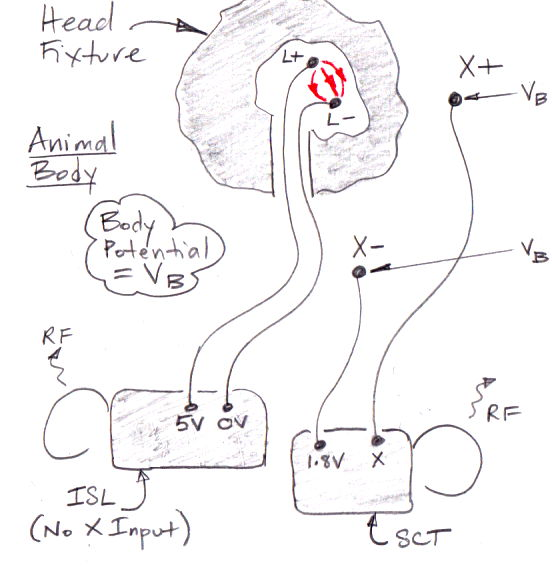Figure: Lamp Current Flowing For Isolated Stimulator and Sensor Circuits. This arrangement of two separate implants proved successful in eliminating lamp artifact. The A3037 is equipped with two batteries and an isolator in order to achieve the same effect.

The MS-ISL is equipped with two batteries. The master circuit communcates with the stimulator through an isolator. The only connection between the two circuits is a 50-pF capacitance between their 0-V potentials. When the stimulator turns on the lamp, the average voltage on the lamp pins drops by 1.8 V with respect to the stimulator 0-V potential, which means the stimulator 0-V potential must drop by 1.8 V with respect to the animal body. We estimate the capacitance between the stimulator and the animal body is dominated by the capacitance of its two stimulus leads. Assuming 50-mm leads, their combined capacitance is 100 pF. Assuming a lead resistance of 25 Ω, the time constant of this charging will be 2.5 ns, which is negligible compared to our shortest sample period.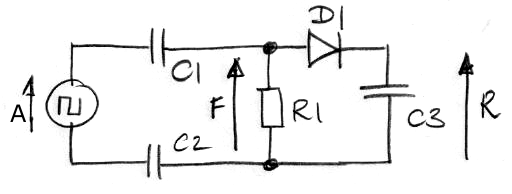Figure: Capacitor-Isolation Test Circuit. We have: applied voltage X, feedthrough voltage F, and rectified voltage R. Components are isolation capacitors C1 and C2, load resistor R1, rectifier D1, and balast capacitor C3.

Meanwhile, the X− lead of the MS-ISL master circuit is connected to the body by a wire and a reference electrode of impedance <10 kΩ. When the stimulator 0-V drops by 2 V with respect the animal body, the 50-pF isolation capacitance will pull the master 0-V potential down by 2 V as well. The 50-pF isolation capacitance then charges through the X− lead its electrode until the 0-V potential of the master is restored to its usual level. Its usual leval is 1.2 V below the potential of the animal body, this being the VCOM potential of the X− electrode. Assuming a reference electrode of impedance <10 kΩ, this charging will take 500 ns, which is negligible compared our shortest sample period.

None yet.

## Development

### Winter-20

[11-FEB-20] We try out the LTV355T optoisolator. We apply a square wave drive current to the LED through a resistor R1, connect the collector directly to 3.7 V, and connect the emitter through a resistor R2 to 0 V. In one test, we have a capacitor C1 = 56 pF in parallel with R2 to minic the gate capacitance of a lamp-switching mosfet.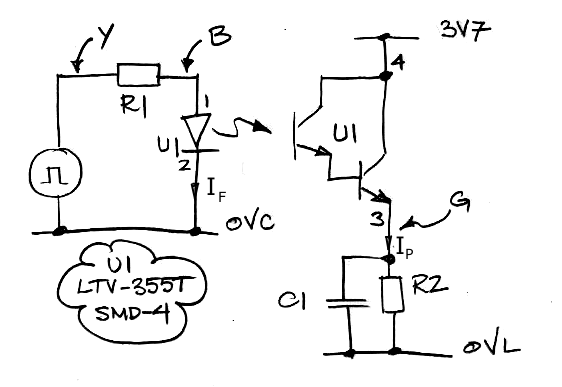Figure: LTV355T Optoisolator Test Circuit. Square wave drive voltage Y, LED voltage B, and photodarlington emitter voltage G, are named after the colors we use to display them on our oscilloscope.

With Y = 2.0 V and R1 = 10 kΩ we see B = 1.0 V, which implies IF = 100 μA. With R2 = 1 kΩ, G rises to 3.0 V. When we add a 56 pF capacitor in parallel with the load resistor and the rise and fall times are unaffected.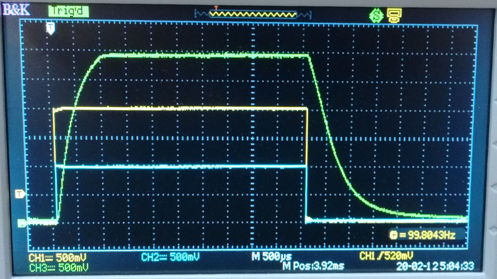Figure: LTV355T Switching Signal. Yellow: Y, Blue: B, Green: G, for R1 = 10 kΩ, R2 = 1 kΩ.

We vary R1, R2, and Y to obtain the folling table of rise and fall times, as well as the emitter voltages attained by the photocurrent running through R1.

Drive Current
(μA)
B
(V)
R2
(kΩ)
Rise Time
(ms)
Fall Time
(ms)
403.01002100
403.010210
401.2111
1003.010.51
1203.010.51
2003.010.20.5
Table: Optoisolator Performance. We give approximate 10-90% rise and fall times. Voltage B is photodarlington emitter voltage, R2 is photodarlington emitter resistor.

With IF = 40 μA, G = 1.2 V, so IP = 1.2 mA and IP/IF = 30 = 3000%. Looking at Fig 5 of the LTV355T data sheet, Current Transfer Ratio versus Forward Current, we expect CTR increasing from 1000% at 100 μA to 4000% at 700 μA. And yet we see 3000% at 40 μA. The data sheet gives minimum and maximum values of CTR at IF = 1 mA of 600% and 7500%.

If we power the LED with an ML1220 battery, the battery's source resistance will be roughly 40 Ω and its voltage will be roughlly 2.5 V, creating a power supply we shall call 2V5. A 100 μA LED drive current will drop 2V5 by 4 mV. Assuming an amplifier gain of 100 and average amplifier output 1.2 V, a 4 mV drop in 2V5 will cause a 40 mV ÷ 100 ÷ 2 = 20 μV rise in the apparent sensor voltage. This rise will be further attenuated by a 1 kΩ resistor and 10 μF capacitor we use to filter 2V5 into the analog supply voltage VA, for a time constant of 10 ms.

[13-FEB-20] Another way to isolate the master and stimulator circuits is with capacitors. We build the following circuit with the help of a 1N34A germanium diode.Figure: Capacitor-Isolation Test Circuit. We have: applied voltage A, feedthrough voltage F, and rectified voltage R. Components are isolation capacitors C1 and C2, load resistor R1, rectifier D1, and balast capacitor C3.

We apply a 0.0-2.5 V square wave to A using a function generator. We measure R with a battery-powered multimeter so we do not compromise our isolation with measurement grounds. We vary the frequency of the square wave.Figure: Rectified Voltage vs Frequency. Blue: C1 = C2 = 10 nF, R1 = 1.0 MΩ, C3 = 100 nF. Orange: C1 = 10 nF, C2 = 0.0 nF, R1 = 1.0 MΩ, C3 = 100 nF. Yellow: C1 = C2 = 10 nF, R1 = 10 kΩ, C3 = 100 nF. Green: C1 = C2 = 10 nF, R1 = 10 kΩ, C3 = 100 pF. Brown: C1 = C2 = 100 pF, R1 = 10 kΩ, C3 = 50 pF. Cyan: C1 = C2 = 100 pF, R1 = 39 kΩ, C3 = 50 pF. Red: C1 = C2 = 200 pF, R1 = 39 kΩ, C3 = 50 pF

With R1 = 1 MΩ, C1 = 10 nF, and C3 = 100 nF, we modulate a 32-kHz square wave so as to view the rise and fall time of R. We must short C2 with our oscilloscope probe ground clips in order to view R.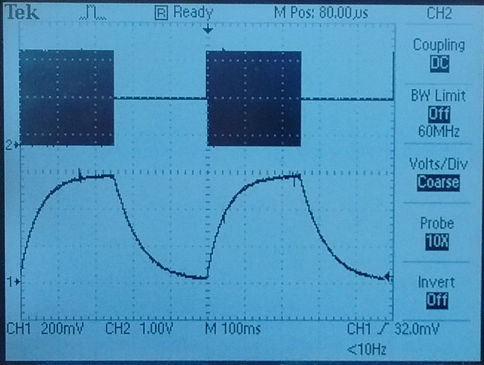Figure: Capacitor-Isolated Voltage Transfer. Top: A, an amplitude-modulated, 32-kHz square wave. Bottom: R. We have C1 = 10 nF, C2 shorted by ground clips, C3 = 100 nF, R1 = 1.0 MΩ.

The 100-ms rise and fall times are consistent with R1C3 = 1.0 MΩ × 100 nF. We drop R1 from 1 MΩ to 10 kΩ and repeat the same measurement with a 32-kHz, modulated square wave.Figure: Capacitor-Isolated Voltage Transfer. Top: A, an amplitude-modulated, 32-kHz square wave. Bottom: R. We have C1 = 10 nF, C2 shorted by ground clips, C3 = 100 nF, R1 = 10 kΩ.

The rise time of 1 ms is consistent with R1C3 = 10 kΩ × 100 nF = 1 ms. The falling slope of 1.0 V in 50 ms is consistent with D1 draining C3 at 2 μA. The 1N34A data sheet gives saturation current 5 μA. With a continuous 32-kHz square wave, we look at F. We must short C2 with our ground clips in order to make this observation. But we confirm with our isolated meter that F has average value zero when capacitor C2 is not shorted.Figure: Capacitor-Isolated Voltage Transfer. Top: A, a 32-kHz square wave. Bottom: F. We have C1 = 10 nF, C2 shorted by ground clips, C3 = 100 nF, R1 = 10 kΩ.

We reduce C3 to 100 pF and keep R1 = 10 kΩ. We are shorting C2 with our oscilloscope ground clips, but we the rectified voltage still rises to 1.5 V, which matches our isolated measurement with battery-powered multimeter. We apply modulated 32-kHz to A.Figure: Capacitor-Isolated Voltage Transfer. Top: A, an amplitude-modulated, 32-kHz square wave. Bottom: R. We have C1 = 10 nF, C2 shorted by ground clips, C3 = 100 pF, R1 = 10 kΩ.

We previously had the balast capacitor much greater than the isolating capacitors. Now we have the balast capacitor much smaller than the isolating capacitors. The rising slope of R is 1.0 V in 10 μs, which is consistent with C3 = 100 pF being charged by a forward-biaset D1 with 10 μA. The falling slope of 1.0 V in 200 μs is consistent with a reverse-biased D1 draining C3 = 100 pF at 2 μA.Figure: Capacitor-Isolated Voltage Transfer. Top: A, an amplitude-modulated, 32-kHz square wave. Bottom: R. We have C1 = 100 pF, C2 shorted by ground clips, C3 = 100 pF, R1 = 10 kΩ.

[14-FEB-20] With C1 = C3 = 100 pF, C2 shorted, and R1 = 10 kΩ, R has rises to 1.3 V, which is half the amplitude of A. We try C1 = C2 = C3 = 100 pF and R1 = 10 kΩ, with the addition of a 1 kΩ current sense resistor in series with the function generator return. At 32 kHz we have R = 1.02 V. The voltage across the current sense resistor is a sequence of alternating spikes 30 mV rms and average value within 500 μV of zero. We increase frequency to 200 kHz to obtain a better view of the spikes, which are now 60 mV rms, implying current 60 μA rms. The time constant of the spikes is consistent with R1 = 10 kΩ charging C3 = 100 pF, which is 1 μs.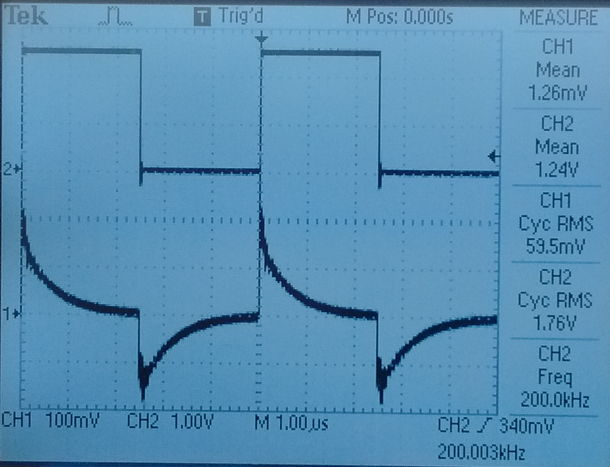Figure: Input Current Spikes. Top: A, a 200-kHz square wave. Bottom: Voltage across 1-kΩ sense resistor. We have C1 = C2 = C3 = 100 pF and R1 = 10 kΩ.

We return to 32 kHz. We have C1 = C2 = C3 = 100 pF and R1 = 10 kΩ plus a 1-kΩ sense resistor. We measure R = 1.02 V with our battery-powered meter, and current 30 μA rms. We remove R1 and R = 0.00 V. We try C1 = C2 = 10 pF, C3 = 100 pF, and R1 = 10 kΩ and get R = 0.25 V and current 6 μV rms. We remove R1 and get R = 0.00 V

The load resistor's function is to discharge the isolation capacitors. On the rising edge of A, 2.5 V appears across the load resistor, and the isolation capacitors charge until they each have 1.25 V across them. During the brief charging period, the diode charges the balast capacitor to 1.25 V. After that, the diode is reverse-biased by roughly 1 V and discharges the balast capacitor slowly. On the falling edge of A, the load resistor discharges the isolation capacitors. The diode continues to discharge the balast capacitor slowly, but the rectified voltage has dropped by only 0.4 V by the time the next rising edge occurs. On the rising edge, because the isolation capacitors are discharged, they now generate a positive feedthrough voltage, which raises the rectified voltage back up to 1.25 V. The result is an average rectified voltage of 1.0 V.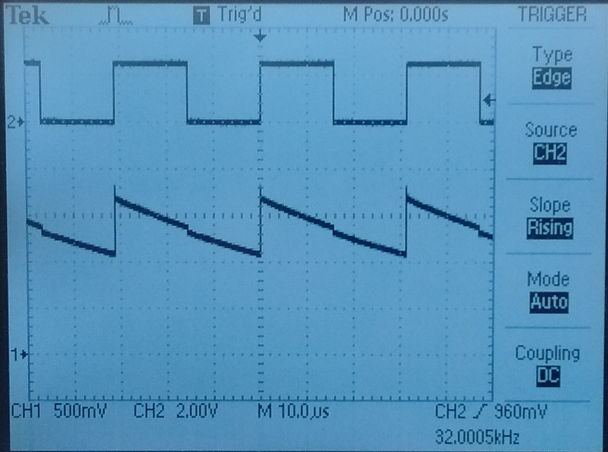Figure: Capacitor-Isolated Voltage Transfer. Top: A, 32-kHz square wave. Bottom: R. We have C1 = 100 pF, C2 shorted by ground clips, C3 = 50 pF, R1 = 39 kΩ..

If we remove the load resistor, the rectification failes. After enough positive cycles of the square wave, the isolation capacitors have charged up to 1.25 V each, due to current through the diode and the 10 MΩ impedance of our meter. They do not discharge on the negative cycles because the diode inhibits current in the dischrage direction. On the positive halves of the square wave, the feedthrough voltage is zero. On the negative halves, the feed through voltage is −2.5 V. The balast capacitor does not discharge to a negative voltage because the diode is reverse-biased.

With C1 = C2 = C3 = 100 pF and R1 = 10 kΩ, each time the 2.5-V square wave transitions, our current sense resistor reports that 125 pC of charge moves in or out of the capacitors. If we deliver the square wave with a logic output, the logic chip power supply will deliver 125 pC on each rising edge, and the logic chip output will drain 125 pC on each falling edge. At 32 kHz, the current drawn from the power supply will be 125 pC × 32 kHz = 4 μA. For this current, we get 1.0 V on C3 = 100 pF, which is sufficient to turn on the DMG1024UV mosfet we use to switch the lamp power in the A3036 stimulator. That mosfet's gate capacitance is 60 pF. We predict that R for 60 pF will be 100 ÷ 60 × 1.0 V = 1.7 V. The rise and fall time of the gate voltage in response to turning on and off the 32 kHz square wave will be around 100 μs and 200 μs respectively, fast enought to generate 500-μs flashes of light.

Our capacitive isolator delivers five times faster response than our opto-isolator, but at 4% of the battery current consumption. It's board area is four P0402 components (for the diode we can use the SMS7630-040LF), or 7 sq.mm. The opto-isolator alone is 29 sq. mm. Of course, the optoisolator offers complete isolation between the two circuits, while the connection between the 0-V potential of the A and R circuits in our capacitive isolator is two 100 pF in series, or 50 pF. But our calculations suggest that this 50 pF is insignificant.

[17-FEB-20] We try C1 = C2 = 100 pF, R1 = 10 kΩ, and C3 = 50 pF and plot frequency response of R. We try R1 = 39 kΩ and repeat. The figure below shows R with C2 shorted. Rise and fall times of order 100 μs, see here. With continuous 32 kHz, the voltage R is shown below, droops by 500 mV, but is never less than 1.1 V. When C2 is not shorted, we presume that the voltage droops by 500 mV, its average is 1.1 V, which means its minimum will be 0.85 V, which is is not sufficient to guarantee that our DMG1024UV will turn on.

We try C1 = C2 = 200 pF, R1 = 39 kΩ, and C3 = 50 pF. At 32 kHz R measured with our DVM is 1.27 V and achieves a maximum at 60 kHz. We plot R versus frequency. This arrangement is sufficient to turn on our mosfet at a cost of 8 μA. For modulated 32 kHz, rise and fall times are 100 μs and 250 μs respectively, as shown below.Figure: Capacitor-Isolated Voltage Transfer. Top: A, an amplitude-modulated, 32-kHz square wave. Bottom: R. We have C1 = 200 pF, C2 shorted by ground clips, C3 = 50 pF, R1 = 39 kΩ..

[18-FEB-20] We assemble an isolator out of SMT components mounted on SIP adaptors. Our C1 = C2 = 200 pF in P0402, our R1 is 50 kΩ in P0402. Our D1 is SMS7621-079LF, and Q1 is the ZVN3306F in SOT-23, connected to an axial resistor and radial white LED. We are using the gate capacitance of Q1 in place of C3. This capacitance is typically 35 pF.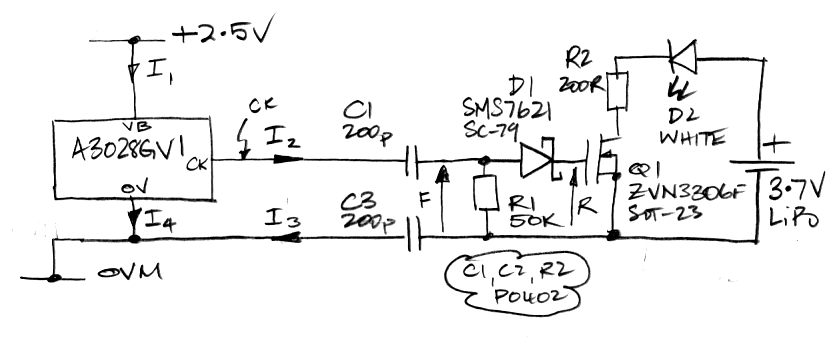Figure: Capacitor-Isolation Surface-Mount Test Circuit. We measure I1. We have I2 = I3, average values of I2 and I3 are zero, and I1 + I2 = I3 + I4.

We produce the 32 kHz, 2.5-V square wave with an A3028GV1 powered by 2.5 V. With CK disconnected, I1 = 92.3μA. We connect CK to the isolator and I1 = 98.9 μA, making isolator current 6.6 μA. The LED turns on. Voltage R is 2.0±0.2 V. Our diode is the SMS7621-079 power detector. We started with the SMS7630-079 zero-biase diode, but its resistance with zero biase is too low, and it discharges the gate capacitance too quickly even when reverse-biased by 1 V.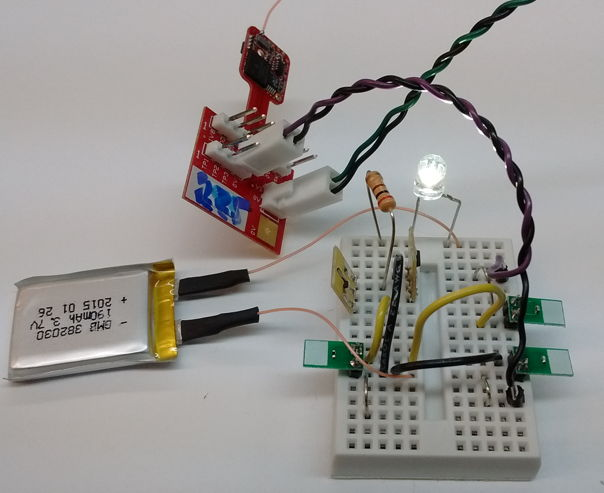Figure: Capacitive Isolator with SMT Components. We have C1 = C2 = 200 pF, R1 = 50 kΩ, D1 = SMS7621-079LF, all mounted on SIP adaptor boards. A LiPo battery powers the lamp. The 32 kHz is delivered by an SCT circuit.

We supply CK now with our function generator and modulate at 1 kHz. We short C2 with our probe grounds so we can look at CK, F, and R at the same time.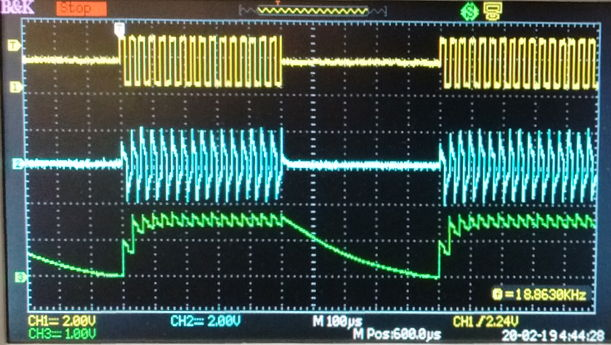Figure: Capacitive Isolation. Showing CK (Yellow), F (Blue), and R (Green). We have C1 = 200 pF, C2 shorted by probe grounds, R1 = 50 kΩ, D1 = SMS7621-079LF.

Rise time from 0.0 V to 1.2 V is 50 μs, and fall time from 1.5 V down to 0.5 V is 200 μs. The DMG1024UV, if we choose to use it in the A3037, has gate capacitance 60 pF and threshold somewhere between 0.5 and 1.0 V.

[24-FEB-20] We place an A3028E-AA SCT in a 500-ml beaker of water with an A3036A IST connected to an A3036IL-A implantable lamp. The SCT's X electrodes are 10 mm apart on the far side of the beaker from the L contacts on the lamp. We flash the lamp at 10 Hz for 10 ms. Artifact on the SCT input is 600μV negative pulses of 10 ms. We cover the lamp with gaffer's tape. Water can pass in and out of the tape wrapper. Artifact is 50 μV positive pulses of 10 ms. We exchange the locations of the X electrodes. Artifact is 40 μV negative pulses of 10 ms. We remove tape and separate the X electrodes by the diameter of the beaker. Artifact is 20-mV positive spikes of 10 ms. We remove SCT from water, hole electrodes in hands and observe heartbeat.